As discussed in the previous articles,  JavaScript follows the ECMAScript (ES) standards. The ES6 or ECMAScript 2015 specifications led to the introduction of some of the most revolutionary features for JavaScript, like Arrow Functions, Classes, Rest and Spread operators, Promises, let and const, etc. In this tutorial, we'll discuss details of Arrow function in JavaScript, which are sometimes also called "fat arrow functions" and represented by the symbol "=>".

• What are the Arrow Functions in JavaScript?
• How to define an Arrow Function in JavaScript?
• What are the everyday Use Cases for Arrow Functions?

## What are Arrow Functions in JavaScript?

An Arrow Function in JavaScript is a syntactically compact option/ alternative to a regular function expression. These are anonymous functions with their unique syntax that accept a fixed number of arguments and operate in the context of their enclosing scope - i.e., the function or other code where they are defined. They are also known as "Fat Arrow" functions, as they utilize a new token, =>, which looks like a fat arrow. So, what makes the Arrow function so unique in JavaScript? Let's take a look at some examples in the following sections to learn and understand the uniqueness of Arrow functions and what motivated to include Arrow functions as one of the primary constructs of the language:

### Concise and small syntax:

Consider the below example of a regular function in JavaScript:

``````// Regular function without arrow

let func1 = function() {
console.log('func');
}
``````

Now, let's use the arrow functions instead and see how it looks.

``````let func1 = () => {
console.log('func')
}
``````

So, we write the code to achieve the same functionality using the Arrow function in a very shorter format. It is one of the main motivations behind introducing the arrow function. It lets you write shorter and more concise code.

### No separate "this" binding:

Before the introduction of the arrow functions, the concept of this was very confusing in JavaScript. The arrow function helps in reducing the confusion around this keyword.

• Note: We will cover the details of "this" keyword and its usage and behavior in the next article about the "this" keyword.

Let's now move forward and discuss how the Arrow Functions can be declared and used in JavaScript:

## How to define an Arrow function in JavaScript?

There are a variety of syntaxes using which one can define Arrow functions in JavaScript. We'll cover the common ones here to get you started. Let's compare how ES5 code with function expressions can now be written in ES6 using arrow functions:

### Declaring Arrow function with Multiple Parameters:

When the function accepts multiple parameters, one can represent it syntactically using Arrow function as follows:

``````(param1, param2, paramN) => { statements to be executed }
``````

Below code snippet shows an example to show the difference between ES5 and ES6 for this syntax:

``````// ES5

var addES5 = function(param1, param2) {
return param1 + param2;
};

// ES6
const addES6 = (param1, param2) => { return param1 + param2 };

``````

As is evident from the above example, the arrow function helps to accomplish the same result with fewer lines of code and approximately half the typing.

If there is only one statement that needs to execute as part of the function, then the curly brackets and the return keyword can be excluded.

Its syntax will look like below:

``````(param1, param2, paramN) => expression to be executed
``````

We can modify the above-written code snippet as below to describe the same without brackets:

``````const addES6 = (param1, param2) => param1 + param2;
``````

Let's understand the details of both these syntaxes with the help of following code snippet:

``````<html>
<body> Demonstrating arrow functions with multiple parameters in javascript:</br>
<script type="text/javascript">
// ES5

{
return param1 + param2;
};

// ES6
const addES6 = (param1, param2) =>
{
var sum  = param1 + param2;
return sum;
};

// ES6 single line
const addSingleExpressionES6 = (param1, param2) =>  param1 + param2;

document.write("Sum using ES5: " + addES5(10,20) + "</br>");
document.write("Sum using ES6: " + addES6(10,20) + "</br>");
document.write("Sum using ES6 Single Expression: " + addSingleExpressionES6(10,20) + "</br>");

</script>
</body>
</html>

``````

Save the file with name arrowFunctionMultiParam.html and open it in any browser (Chrome, Firefox, or IE). It should show the output as: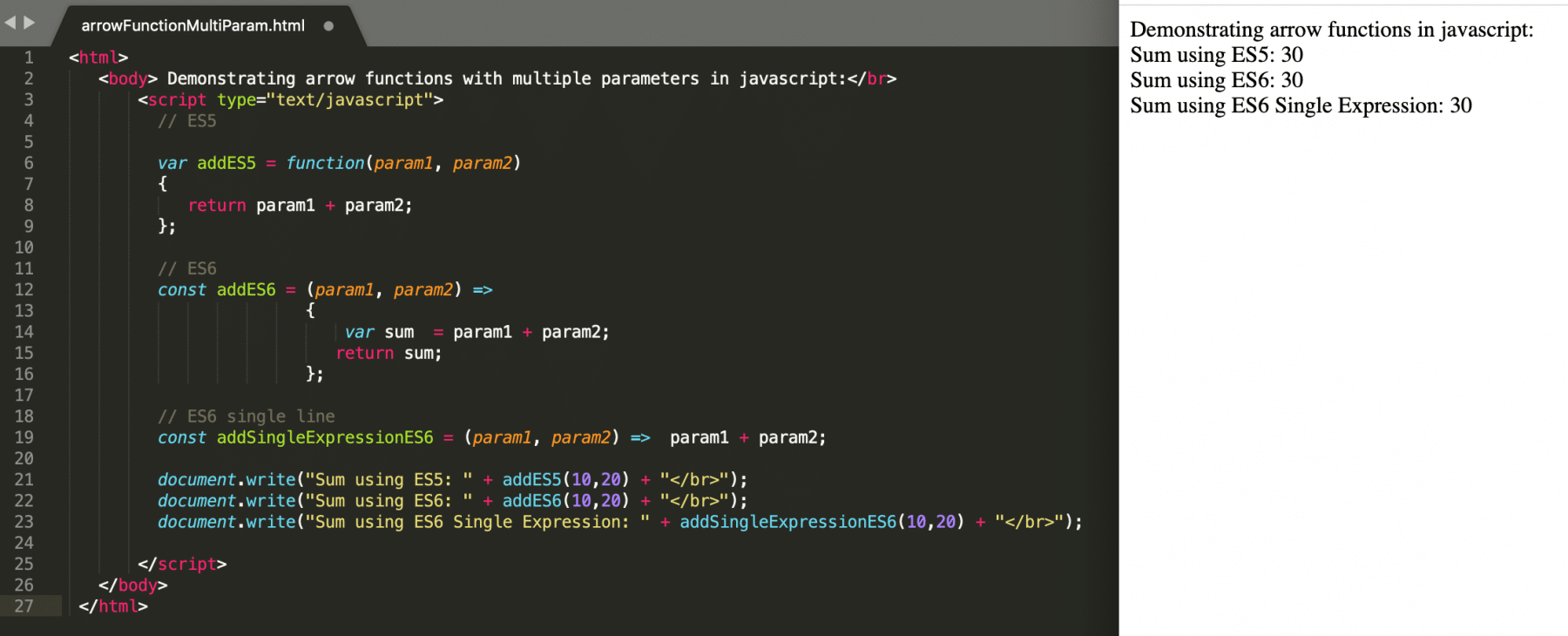As we can see from the above screenshot, we can achieve the same task using the arrow function (=>) in a very compact code format.

### Declaring Arrow function with One Parameter:

When there is only one parameter, even the parenthesis of the parameter is optional. Its syntax looks like below:

``````// If multiple statements need to be executed
param1 => { statements to be executed }

// If only one expression need to be executed
param1 => expression to be executed
``````

Consider an example where the user needs to split a string and the only parameter that will pass to the function which needs to split. Let's understand how a user can use the Arrow function to achieve the same and what will be the difference if we need to accomplish the same task using the ES5 function expressions.

``````<html>
<body> Demonstrating arrow functions with single parameter in javascript:</br>
<script type="text/javascript">

//ES5
var splitResultEs5 = function stringSplitter(inputString) {
return inputString.split(' ');
};

//ES6 : No paranthesis around the parameter "inputString"
const splitResultEs6 = inputString => inputString.split(" ");

document.write("Split result using ES5: " + splitResultEs5("This is ToolsQA Demo") + "</br>");
document.write("Split result using ES6: " + splitResultEs6("This is ToolsQA Demo") + "</br>");

</script>
</body>
</html>

``````

Save the file with name arrowFunctionSingleParam.html and open it in any browser (Chrome, Firefox, or IE). It should show the output as: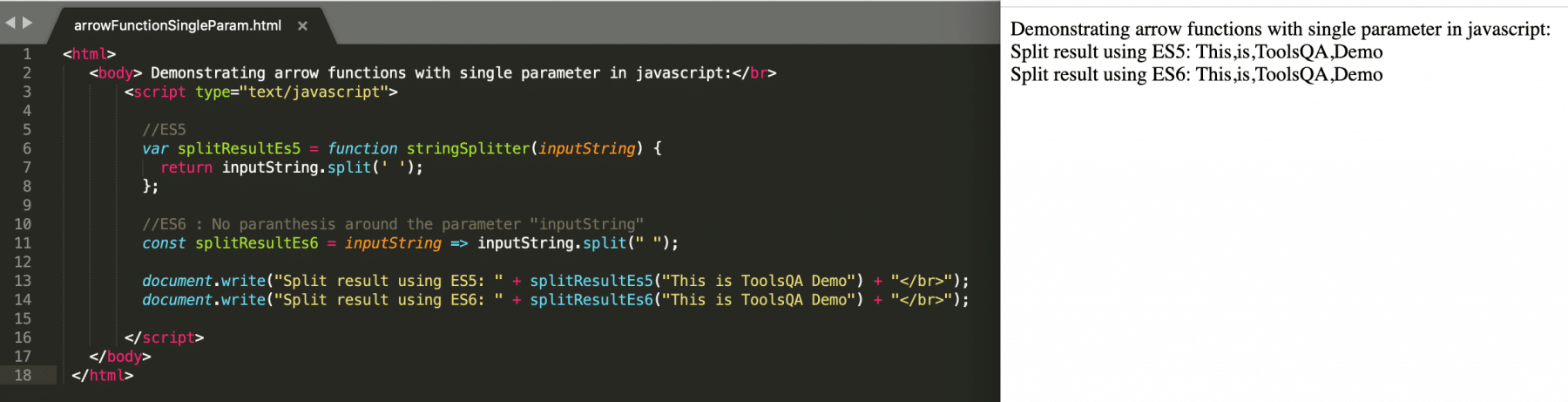As is evident from the above screenshot, while declaring the Arrow function, no specification of the parenthesis happened for the parameter "inputString", and the overall code was much slick compared to the ES5 syntax.

### Declaring Arrow function with No Parameter:

When the function doesn't accept any parameters, then the parenthesis is mandatory. Its syntax looks like below:

``````// If multiple statements need to be executed
() => { statements to be executed }

// If only one expression need to be executed
() => expression to be executed
``````

Let's see how to declare and use an Arrow function which doesn't accept any parameters with the help of following code snippet:

``````<html>
<body> Demonstrating arrow functions with no parameter in javascript:</br>
<script type="text/javascript">

//ES5
var printEs5 = function print() {
document.write("This is ToolsQA demo for Arrow functions</br>");
};

//ES6 : No paranthesis around the parameter "inputString"
const printEs6 = () => document.write("This is ToolsQA demo for Arrow functions</br>");

printEs5();
printEs6();

</script>
</body>
</html>
``````

Save the file with name arrowFunctionNoParam.html and open it in any browser (Chrome, Firefox, or IE). It should show the output as: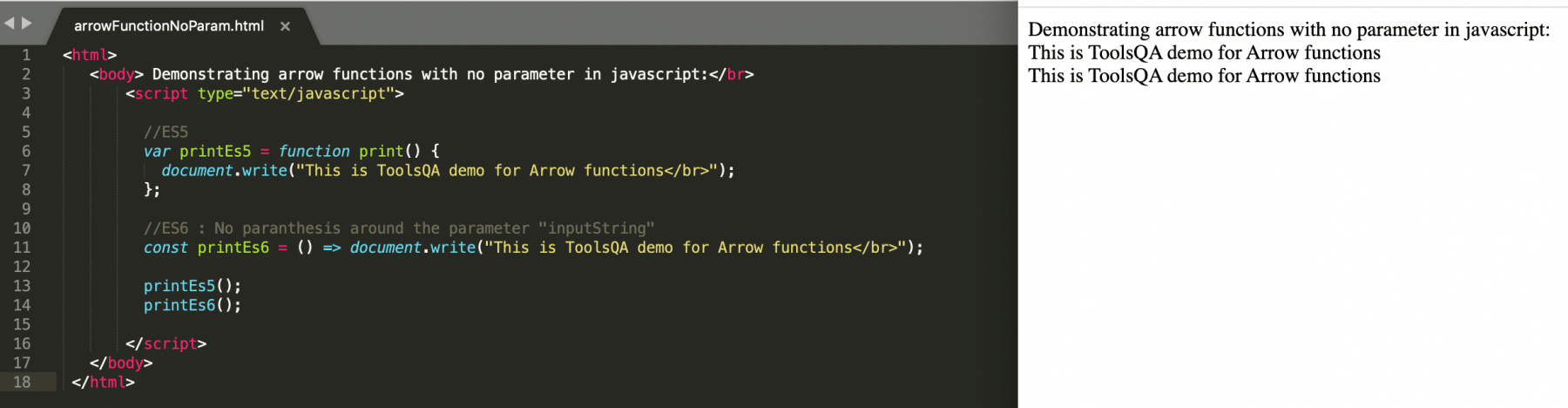As we can see from the above screenshot that mentioning parenthesis is mandatory when the Arrow function is not expecting any parameters.

### Declaring Arrow function with Object Literals:

Arrow functions can also return an object literal expression. Its syntax looks like below:

``````(param1, param2) => ({ var1: param1, var2: param2 });
``````

The main thing to note here is that the return body needs to wrap in parentheses to distinguish between a block and an object, as both of them use curly brackets.

Let's see how to declare and use an Arrow function which returns an object with the help of following code snippet:

``````<html>
<body> Demonstrating arrow functions which returns an object in javascript:</br>
<script type="text/javascript">

//ES5
var returnObjectEs5 = function returnObject(param1, param2) {
return {
var1: param1,
var2: param2
};
};

// ES6
var returnObjectEs6 = (param1, param2) => ({ var1: param1, var2: param2 });

console.log("Return an object in ES5: ");
console.log(returnObjectEs5(1, "Lakshay"));
console.log("Return an object in ES6: ");
console.log(returnObjectEs6(2, "Lakshay"));

</script>
</body>
</html>
``````

Save the file with name arrowFunctionReturnObject.html and open it in any browser (Chrome, Firefox, or IE). It should show the following output in the browser's console: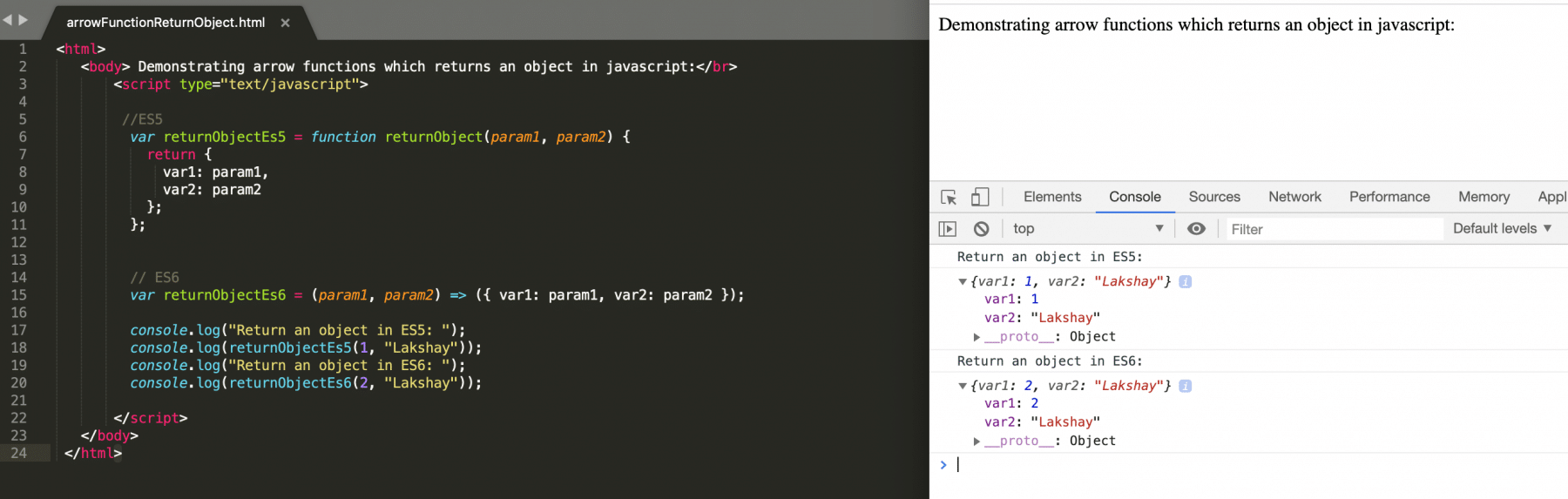As is evident from the above screenshot, the Arrow function returns an object. The only condition is it needs to wrap in the parenthesis.

## What are the common Use Cases for Arrow Functions?

Arrow functions can prove helpful where the developer wants to reduce the overall code structure and want to write minimal code. Let's discuss a few of the practical use-cases where Arrow functions have proved their worth:

• Array manipulations using map/reduce/filter functions
• Promises and callbacks

Let's discuss all of these use cases in detail:

### Using Arrow functions in Array manipulation functions:

One of the very common use case of "Arrow functions" is in the array manipulation functions ".map()/.reduce()/.filter()".

Consider the following ES5 based code snippet to see an example of these array manipulation functions:

``````<html>
<body> Demonstrating Map Reduce and Filter functions using ES5 in javascript:</br>
<script type="text/javascript">

// Usage of Map Function
var inputArray  = [9,3,7,21,6,8];
var outputArray = inputArray.map(function(x) { return x+50 });

document.write("Input Array for Map Function: " + inputArray + "</br>");
document.write("Map function output: " +  outputArray + "</br> </br>");

// Usage of reduce function
var inputArray = [500, 200, 100, 100];

function reduceFunction(firstValue, restTotalValue)
{
return firstValue - restTotalValue;
}

var outputArray = inputArray.reduce(reduceFunction); // Perform reduce on each of the elements

document.write("Input Array for Reduce Function: " + inputArray + "</br>");
document.write("Reduce function output: " +  outputArray + "</br> </br>");

// Usage of filter function
var inputArray  = [2,3,4,5,6,7,8];

var outputArray = inputArray.filter(function(x) { return x%2==0 }); // Will save value to output array
// only when it is divisible by 2

document.write("Input Array for Filter Function: " + inputArray + "</br>");
document.write("Filter function output: " +  outputArray);

</script>
</body>
</html>

``````

Save the file with name mapReduceFilterES5.html and open it in any browser (Chrome, Firefox, or IE). It should show the following output: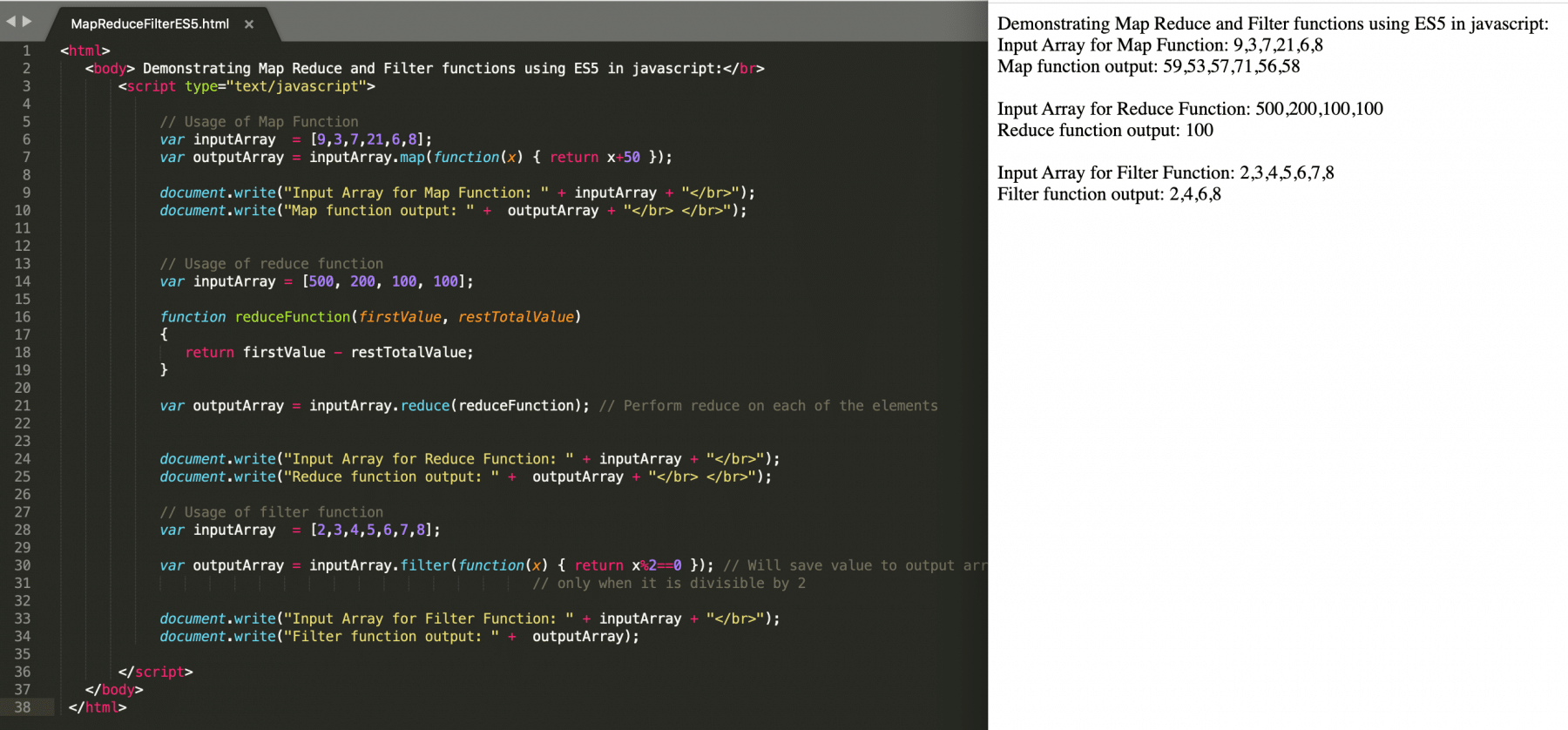Now the same can be re-written with the help of Arrow (=>) functions as below:

``````<html>
<body> Demonstrating Map Reduce and Filter functions using ES6 Arrow Functions in javascript:</br>
<script type="text/javascript">

// Usage of Map Function
var inputArray  = [9,3,7,21,6,8];
var outputArray = inputArray.map(x => x+50);

document.write("Input Array for Map Function: " + inputArray + "</br>");
document.write("Map function output: " +  outputArray + "</br> </br>");

// Usage of reduce function
var inputArray = [500, 200, 100, 100];

var outputArray = inputArray.reduce(x = (firstValue, restTotalValue) => firstValue - restTotalValue); // Perform reduce on each of the elements

document.write("Input Array for Reduce Function: " + inputArray + "</br>");
document.write("Reduce function output: " +  outputArray + "</br> </br>");

// Usage of filter function
var inputArray  = [2,3,4,5,6,7,8];

var outputArray = inputArray.filter(x => x%2==0); // Will save value to output array
// only when it is divisible by 2

document.write("Input Array for Filter Function: " + inputArray + "</br>");
document.write("Filter function output: " +  outputArray);

</script>
</body>
</html>
``````

Save the file with name mapReduceFilterES6ArrowFunctions.html and open it in any browser (Chrome, Firefox, or IE). It should show the following output: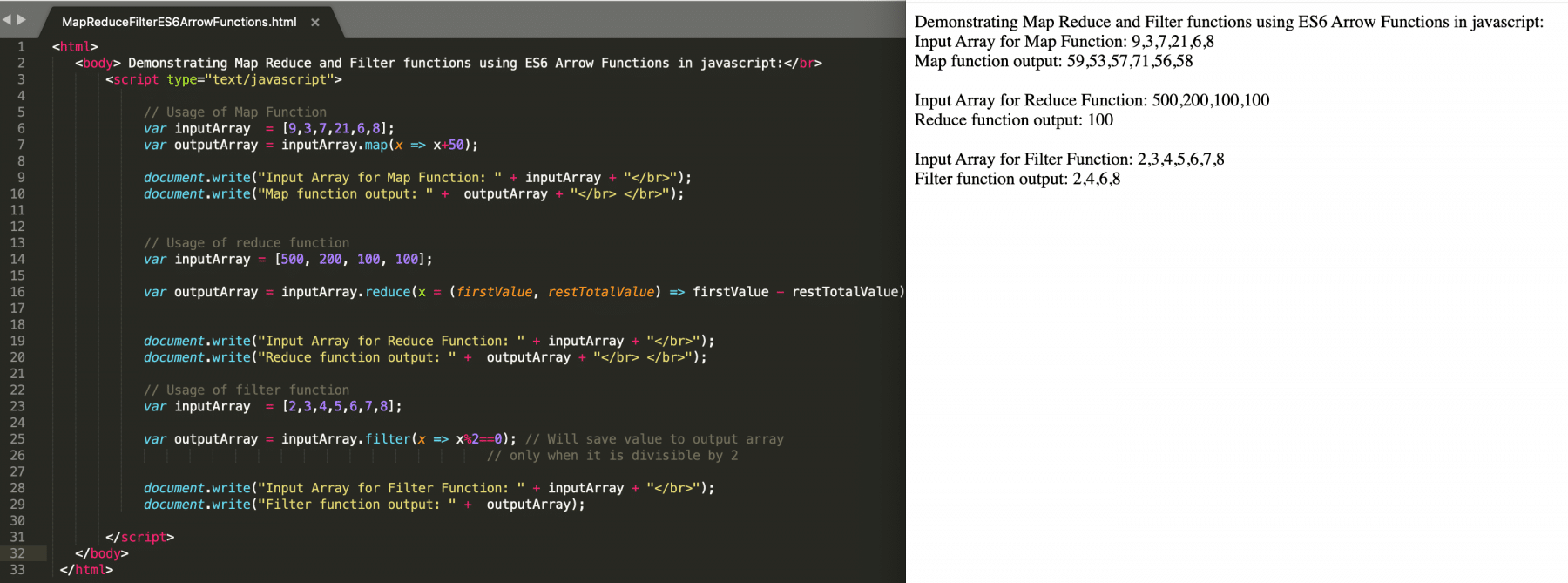As is evident from the above screenshot, the same task of the map, reduce and filter can be achieved with a much cleaner syntax with the help of "Arrow" functions.

### Using Arrow functions in Promises and callbacks:

We know that the asynchronous callbacks or promises contain a great deal of "function" and "return" keywords.

When using promises, we generally use function expressions to chain these calls as follows:

``````<html>

<body> Demonstrating promise consumer using .then()  method in javascript:</br>
<script type="text/javascript">
function createDivAndAppendText(divID, text)
{
const outPutElem = document.createElement("div"); outPutElem.setAttribute("id", divID);
document.body.appendChild(outPutElem); document.getElementById(divID).innerHTML = text + "</br>";
}

function i_take_10_sec(callback)
{
return new Promise((resolve, reject)=>{
setTimeout(() => {
createDivAndAppendText('first', 'I was no: 1 and I take 10 seconds')
resolve('done');
}, 10000);
})
}

function i_take_1_sec(callback)
{
return new Promise((resolve, reject)=>{
setTimeout(() => {
createDivAndAppendText('second', 'I was no: 2 and I take 1 second')
resolve('done');
}, 1000);
})
}

function run()
{
i_take_10_sec()
.then(function(result)
{
return i_take_1_sec();
})
.then(() => {
createDivAndAppendText('done', 'Done')
})
}

run();

</script>
</body>

</html>
``````

Save the file with name promiseThenChaining.html and open it in any browser (Chrome, Firefox, or IE). It should show the following output: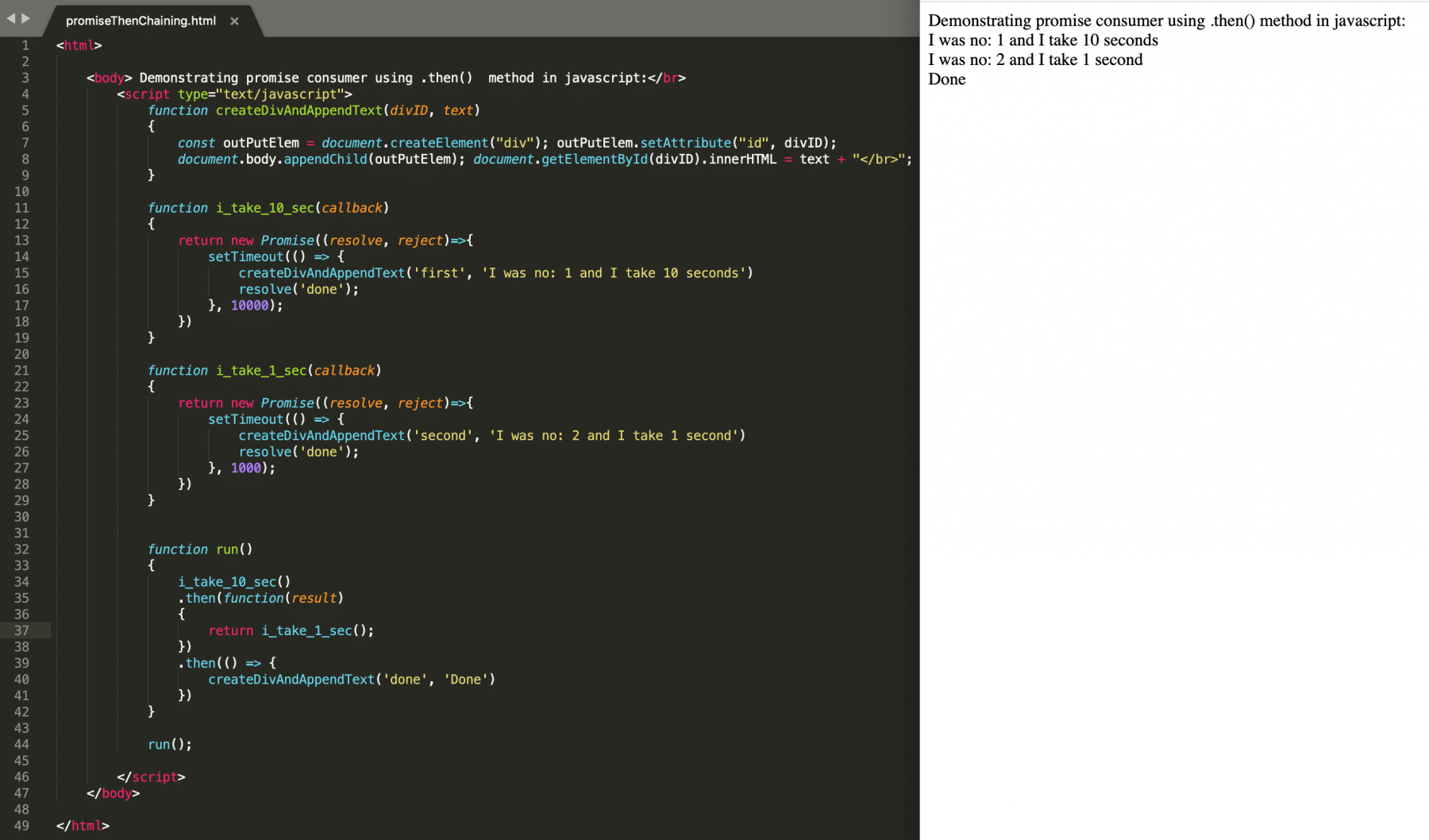One can achieve the same functionality by modifying the above-written program using the Arrow functions as below:

``````<html>

<body> Demonstrating promise consumer using .then()  method in javascript:</br>
<script type="text/javascript">
function createDivAndAppendText(divID, text)
{
const outPutElem = document.createElement("div"); outPutElem.setAttribute("id", divID);
document.body.appendChild(outPutElem); document.getElementById(divID).innerHTML = text + "</br>";
}

function i_take_10_sec(callback)
{
return new Promise((resolve, reject)=>{
setTimeout(() => {
createDivAndAppendText('first', 'I was no: 1 and I take 10 seconds')
resolve('done');
}, 10000);
})
}

function i_take_1_sec(callback)
{
return new Promise((resolve, reject)=>{
setTimeout(() => {
createDivAndAppendText('second', 'I was no: 2 and I take 1 second')
resolve('done');
}, 1000);
})
}

function run()
{
i_take_10_sec().then(result => i_take_1_sec()).then(() => createDivAndAppendText('done', 'Done'));
}

run();

</script>
</body>

</html>
``````

Save the file with name promiseThenChainingWithArrowFunctions.html and open it in any browser (Chrome, Firefox, or IE). It should show the following output: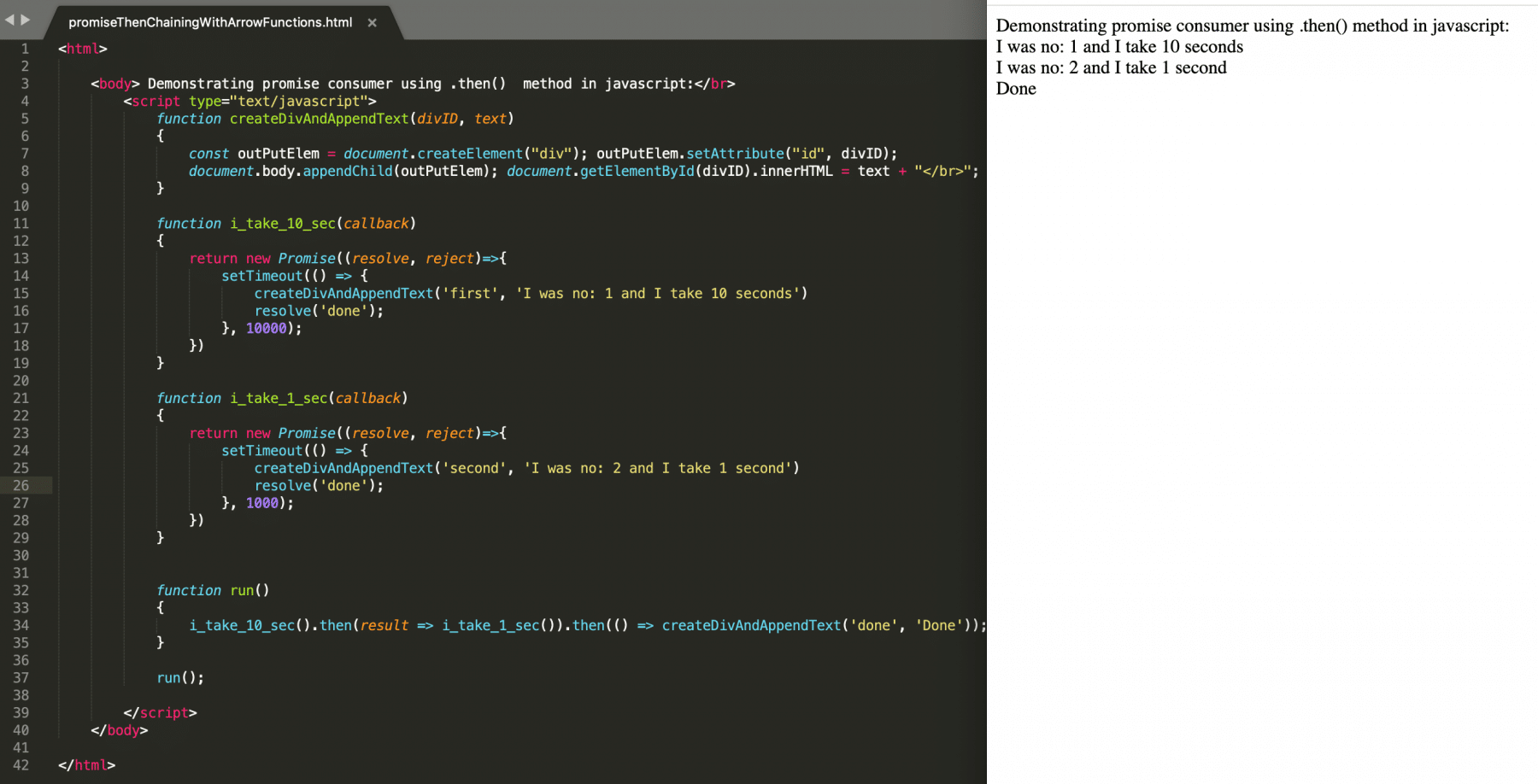So, we can see that the syntax becomes much cleaner and understandable when invoked using the Arrow functions.

## Key Takeaways:

• Arrow functions are one of the most used features of JavaScript and help the developers to achieve the same task with very minimal code.
• Arrow functions provide various different syntax for "no parameter", "one parameter" and "more than one parameter" usage.
• For the single statement functions, the user can skip the return statement in Arrow functions, and the last expression will return implicitly from the Arrow function.
• Arrow functions are the best fit for Array manipulation functions(map/reduce/filter) and with the callback functions.

Let's now move to the next article to understand the concept of this in JavaScript.